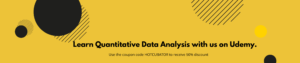Data analysis is a vast ocean and it is not surprising to know that many people feel confused as to what type of statistical test should be undertaken to analyse their data project. There are two types of statistical tests or methodologies that are used to analyse data – Parametric test is more popular and considered to be more powerful statistical test between the two methodologies. The difference between the two tests are largely reliant on whether the data has a normal or non-normal distribution.

#### Parametric tests

The parametric test make certain assumptions about a data set; namely – that the data are drawn from a population with a specific or normal distribution. It is further assumed in parametric test that the variables in the population are measured based on an interval scale.

#### When parametric tests are used

• When the data has a normal distribution
• When the measurement scale is interval or ratio

Types of Parametric test–

• Two-sample t-test
• Paired t-test
• Analysis of variance (ANOVA)
• Pearson coefficient of correlation#### Non-parametric test

Non-parametric test are also known is distribution-free test is considered less powerful as it uses less information in its calculation and makes fewer assumption about the data set.

#### When non-parametric tests are used

• When the study is better represented by the median
• When the data has a normal distribution
• When there is ordinal data, ranked data, or outliers can’t be removed
• When the sample size is very small
• When the measurement scale is nominal or ordinal

Types of Non-parametric test

• Kruskal-Wallis test
• Spearman’s rank correlation
• Wilcoxon signedrank test
• Wilcoxon ranksum test

Here is a basic difference table between the two –